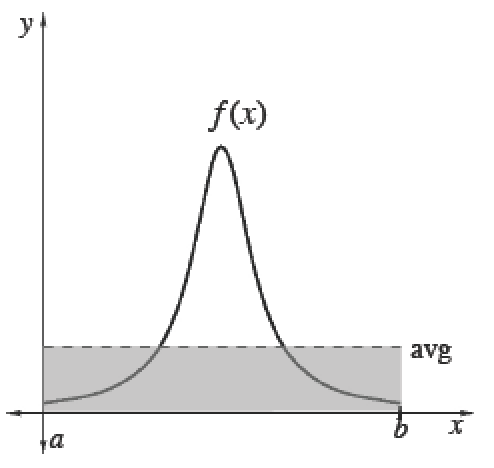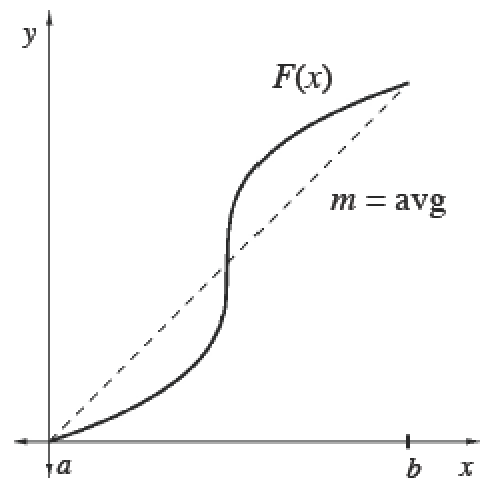### Home > APCALC > Chapter 7 > Lesson 7.3.5 > Problem7-156

7-156.

In order to calculate the average value of a function, sometimes it makes sense to integrate while other times the slope of a secant line is determined. When do you need to use each strategy?

When trying to find the average value of $f\left(x\right)$, the strategy depends on what function is given: $f(x)$ or $F(x)$.

Average (Mean) Values

 To calculate the mean (average) value of a finite set of items, add up the values of items and divide by the number of items.Integrals help us add over a continuous interval. Therefore, for any continuous function $f$ :$\frac{\int_a^bf(x)dx}{b-a}=$ mean value of $f$ over $[a, b]$Since $\int _ { a } ^ { b } f ( x ) d x$, we can also calculate the average value of any function $f$ using its antiderivative $F$. Its average slope gives the average rate of change of $F$, which is the same as the average value of $f.$$\frac{\int_a^bf(x)dx}{b-a}=\frac { F ( b ) - F ( a ) } { b - a }=$ mean rate of change of $F ′$ over $[a, b]$